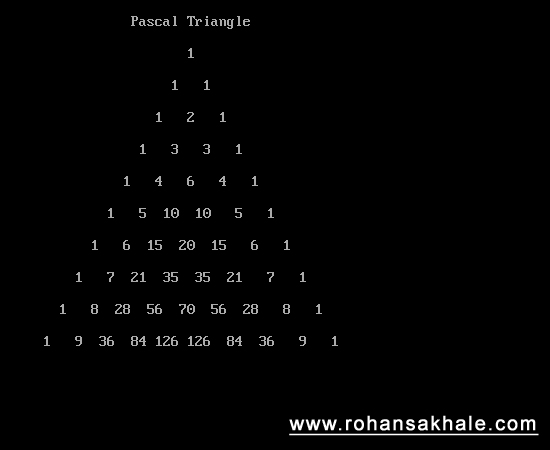# Pascal Traingle

2/7/2012 code-examplecpp

### # Summary

In mathematics, Pascal's triangle is a triangular array of the binomial coefficients in a triangle. It is named after the French mathematician, Blaise Pascal in much of the Western world, although other mathematicians studied it centuries before him in India, Greece, Iran, China, Germany, and Italy. The rows of Pascal's triangle are conventionally enumerated starting with row n = 0 at the top. The entries in each row are numbered from the left beginning with k = 0 and are usually staggered relative to the numbers in the adjacent rows (read more (opens new window))

### # Screenshot### # Code

``````#include "iostream.h"
#include "stdio.h"
#include "conio.h"

void main()
{
int p;
int i,j,k;
clrscr();
cout << endl << "\t\tPascal Triangle" << endl << endl;
for(i=0;i < 10;i++)
{
j = 1;
p[i] = 1;
p[i][i] = 1;
while(j < i)
{
p[i][j] = p[i-1][j-1] + p[i-1][j];
j++;
}
}
for(i=0; i < 10; i++)
{
for(j=10; j > i; j--)
{
cout << "  ";
}
for(k=0; k <= i; k++)
{
printf("%4d",p[i][k]);
}
cout << endl << endl;
}
getch();
}
``````
1
2
3
4
5
6
7
8
9
10
11
12
13
14
15
16
17
18
19
20
21
22
23
24
25
26
27
28
29
30
31
32
33
34
35